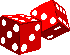dice-playWhat's new...Register and become a memberHomeCrapsGamesOddsShooting/RollingMagic/Psychic DiceTypes of DiceHistoryCollectingLinks & MoreTake a look at some related websites on other gaming topics.DominoesBackgammonLucky DrawsStandard Dice Notation

The number of faces on a die and the number of dice used in a game are conventionally notated as a short sequence of characters.

Regular six-sided dice are notated as d6 and eight-sided dice are d8. The d represents the die. The number of sides on the die is represented by the number following the d.

If you're throwing more than one die, then precede the letter d with the number of dice you're using. So three dice that are six-sided are notated as 3d6 and four dice that are ten-sided are notated as 4d10. The number of dice used is represented by the number preceding the d.

The notation can be modified with a mathematical operator and number.  So 2d6 - 3 means two six-sided dice totalled and then subtracted by 3. The operator could be either: -, +, x, /.

XdY [-] [+] [x] [/] N

X dice with Y-sides operated on by (-, +, x, /) with number N.

A second roll of different dice could be used instead of a fixed operating number.

XdY [-] [+] [x] [/] AdB

The first roll of X dice with Y-sides is operated on by (-, +. x, /) with a second roll of A dice with B-sides.

d% represents a percentile dice. That is one with 100 faces. Two ten-faced dice numbered 0 - 9 and 00 - 90 can be used as percentile dice.  Two ten-faced dice numbered 0 to 9 can be used with one die representing 10s and the other representing units. If both dice are rolled 0 then the roll represents 100%.  Twenty-faced dice are sometimes numbered 0 to 9 twice and can be used as percentile dice in the same way.

Polyhedral solids with semi-regular sides are notated as the number of sides of the regular shaped faces around any vertex (corner). So if a vertex is composed of a triangle and two octagons we notate it as (3, 8, 8). A vertex composed of a triangle and two hexagons is notated as (3, 6, 6).

Copyright © 2022 Stormdark I.P. and Media.  All rights reserved.  www.dice-play.com  This site is for personal use only and content may not be copied or reproduced in any form for any purpose.  Terms & Conditions   Advertising